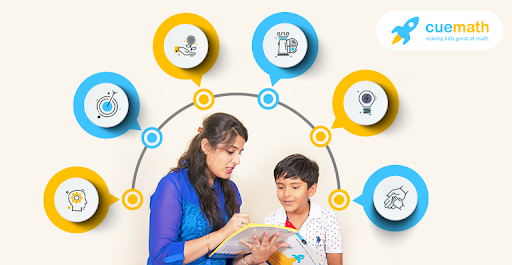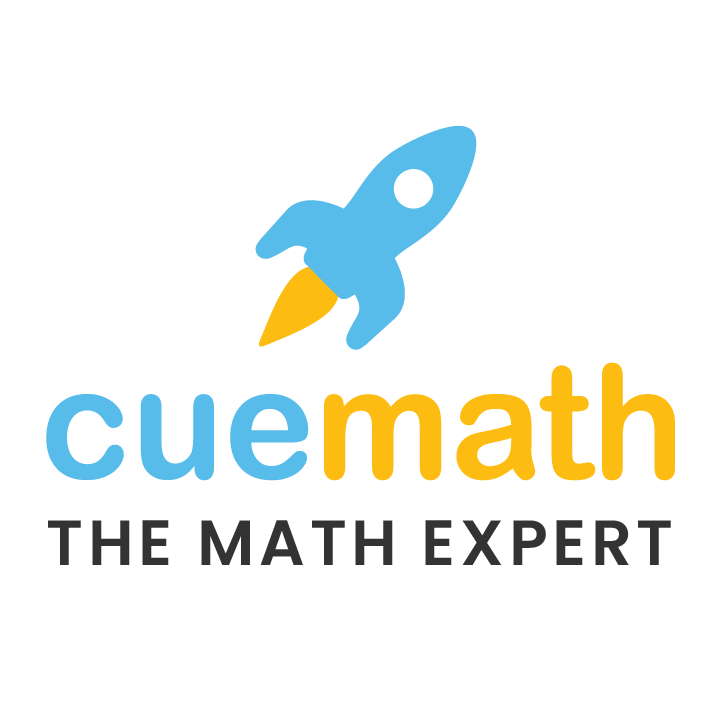# Cuemath vs Vedic Math

3.1k views

 1 Vedic Math – Fast Mental Calculations 2 Cuemath – Learn Math by Reasoning 3 Vedic Math vs Cuemath 4 Comparison of Vedic Math vs Cuemath 5 Conclusion 6 Frequently Asked Questions (FAQs)

18 November 2020

Vedic math has increased in popularity in recent years. Here’s a short article that helps you understand what Vedic Math offers and how it compares with Cuemath.

## Vedic Math – Fast Mental Calculations

Vedic Math is a collection of 16 principles (or patterns) identified and documented in the 1960s. Once memorized, these principles allow one to calculate certain problems mentally.

A combination of these principles can be used to calculate the product of 2 and 3 digit numbers, find the squares of numbers mentally and also calculate cubes and cube roots without long calculations.

Some answers to algebra, calculus and trigonometry questions can also be calculated quickly and mentally using the principles of Vedic math.These techniques can be learned by most students aged 9 and higher. Seeing someone calculate using these 16 Vedic math principles can be quite fascinating. It almost feels like magic. Imagine someone asks what is 105 x 105 and within a second you answer 11025. But it is important to note that these tricks help only in calculations.

Other aspects of math like conceptual understanding, applications and problem-solving are not covered by Vedic Math.

Additionally, most of the tricks used for calculating fast can be memorized from one of the many books available on Amazon or Flipkart. For older students, they can simply learn these tricks from Youtube videos. But neither the classes, not books nor videos will build conceptual understanding.

Vedic math teaches shortcuts to calculate fast not the basics of a concept.

## Learn Math by Reasoning at Cuemath

Cuemath is a math program for children aged 5 to 16. Cuemath focuses on building a strong math foundation and helping children learn conceptually instead of through memorization. It focuses on mental calculations as well as on all math concepts that children learn at school.

Children learn the concepts by solving problems that require them to reason and figure things out.

At the core of the Cuemath learning philosophy is the Cuemath Method. A core principle of this method is learning by reasoning. Cuemath helps children develop their ability to analyze and solve complex problems through reasoning.

Another core principle is conceptual learning by introducing every math concept either via an activity or through pictorial models. Then, Cuemath teachers are trained to help students by not giving the answers away. Instead, they cue the answer by asking leading questions, giving hints so that students discover concepts.All students work on their own learning resources allowing them to speed up or slow down based on their comfort with the topic. Along with this focus on the basics of concepts, students also get sufficient practice to ensure they solve questions efficiently.

Apart from this, students are assigned skill-building exercises on the tab in each class. They are also assigned a math and logic puzzle at the end of every class.

As a parent, if your objective is a strong math foundation across all topics and not just arithmetic, then the Cuemath program is a better choice.

## Vedic Math vs Cuemath

Calculating faster is an impressive skill, however, it is increasingly an outdated skill. Today each one of us carries a powerful smartphone that can perform calculations for us. What is needed today is the ability to figure out how to solve complex unsolved problems. This requires reasoning and analytical skills.

Calculating fast certainly helps in competitive exams (especially if calculators are not allowed). However, only being able to calculate fast but not knowing which calculation to perform in the first place is a common reason for poor performance.

Given a data set and a problem, knowing if one needs to calculate the mean or the median is important. Once this decision is taken any smartphone can calculate the value in an instant.

So students must learn to make good decisions rather than calculate faster. This is where a program like Cuemath is more helpful and will help prepare students better.

## Comparison of Vedic Math vs CuemathVedic MathCuemath

Age group 6+ years 5 to 16-year-olds
Math topics covered Mental calculations only All topics covered by the school
Batch sizes Varies from class to class 6 students per batch
Math learning aids No Abacus, Base-10 blocks, Geo solids, Pattern blocks, Fraction shapes, and 10 more
Aptitude exercises No Yes, every class
Math & logic puzzles No Yes, every class
Core philosophy Calculate faster by memorizing 16 Vedic principles and using them to solve specific questions. Learn by reasoning and build a solid math foundation. Know the reason behind the steps.

## Conclusion

Vedic math is a great technique for mastering mental calculations. However calculating faster using Vedic mathematics tricks, it fails to make a student understand the concepts, applications, and real-life scenarios of those particular problems.

Here comes the role of Cuemath, where the core principle is conceptual learning by introducing every math concept either via an activity or through pictorial models.

Cuemath focuses on building a strong math foundation and helping children learn conceptually instead of through memorization. It focuses on mental calculations as well as on all math concepts that children learn at school.

Cuemath, a student-friendly mathematics and coding platform, conducts regular Online Live Classes for academics and skill-development, and their Mental Math App, on both iOS and Android, is a one-stop solution for kids to develop multiple skills. Understand the Cuemath Fee structure and sign up for a free trial.